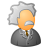Announcements
The way to achieve your own success is the willingness to help somebody else. Go for it!
cancel
Showing results for
Did you mean:Creator

## RangeAvg with 2 variables not calculates

Hi, could anyone pls. help me out, I found a workaround but this would be nicer:

RangeAvg(20, 40)  is the definition or my variable in Qlik sense, it works

But with a nested expression it does not work, can anyone suggest pls why?

RangeAvg

\$(ePDCA_WSAuslief_Pkd_Total(01)), \$(ePDCA_WSAuslief_Pkd_Total(02))

)

Those 2 expressions are complicated enough... how could I pass their value to RangeAvg?????

Thanks a lot! Enikö

12 RepliesMVP

I don't see a reason why this shouldn't work with complicated expression.Where are you trying this? In a chart or text box object?Creator
Author

I try to show the result in both text object:  'Avg.Pkd/Year - Delivery: ' & num(\$(ePDCA_Avg), '# ##0,00')

and also in a Line chart (with YM as Dim) as a Reference Line: =\$(ePDCA_Avg)

Those 2 expressions have Total qualifier in them, to disregard the Line chart's YM dim.

EniköEmployee

Which values \$(ePDCA_WSAuslief_Pkd_Total(01)) and \$(ePDCA_WSAuslief_Pkd_Total(02)) return?Creator
Author

They give the result of this:

RangeSum(

sum(TOTAL{\$<Version={'\$(=Only(Version))'}, Year={'\$(vYear)'}, Month#={"\$1"}, YM= >} Measure1 + Mesure2)

/

(sum(TOTAL{\$<Version={'\$(=Only(Version))'}, OrigVersion={'ACTUAL'}, Year={'\$(vYear)'}, Month#={"\$1"}, YM= >} Measure3) + sum(TOTAL{\$<Version={'\$(=Only(Version))'}, Year={'\$(vYear)'}, Month#={"\$1"}, YM= >} Measure4))

01 for January, 02 for February

Values, like: 6,7 .... 8,9.... 10,5   ...sg in this range.

They return OK values in a text box.

EEmployee

They return single values?

So your rangesum would be rangesum(val1, val2) or they return a list of values?Creator
Author

Each of the 2 above expressions return 1-1 single value (e.g. 7,5 and 8,5) - and I want their avarage.

(((Rangesum is used only for this: if the denominator is zero, (sum of Measure 3+4) the Expressions returns null(). Rangesum turns it into a 0. But lets forget the rangesum, it is not relevant for my problem.)))

Thanks for looking into it, by the wayEmployee

Do they return values with "," ?Partner - Creator II

Hi,

Just a hunch, forgive me if am way off.

sum(TOTAL{\$<Version={'\$(=Only(Version))'}, Year={'\$(vYear)'}, Month#={"\$1"}, YM= >} Measure1 + Mesure2)

(

sum(TOTAL{\$<Version={'\$(=Only(Version))'}, Year={'\$(vYear)'}, Month#={"\$1"}, YM= >} Measure1)

+

sum(TOTAL{\$<Version={'\$(=Only(Version))'}, Year={'\$(vYear)'}, Month#={"\$1"}, YM= >} Mesure2)

)

What if one of the measures has a Null value and is resulting in the Measure1+Measure2 becoming Null.Creator
Author

Hi, sorry I was hold up in a project...

Yes, they do.... so you probably refer to the fact that values have a comma in them, and at the same time tthe separator is a comma....

RangeSum(30,5 , 40,5) does not work but RangeSum('30,5' , '40,5') does calculate.

I just have to figure out who to pass my eExpressions 🙂Tags
Community Browser